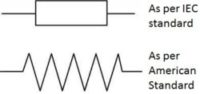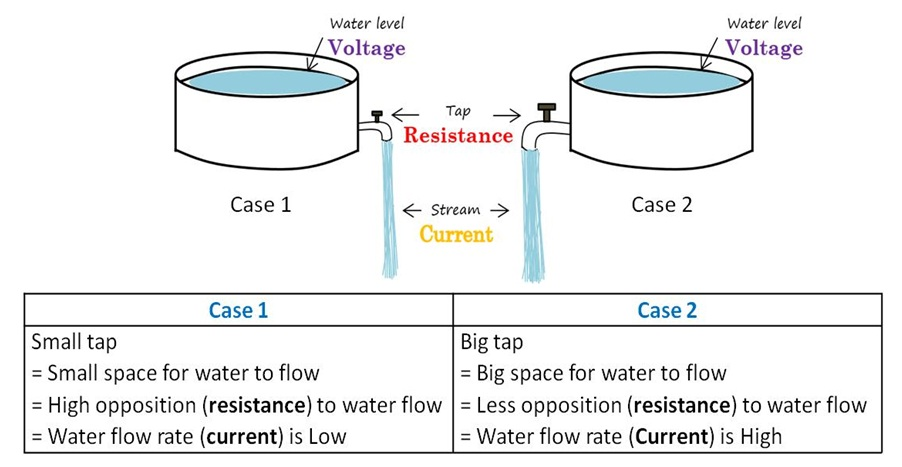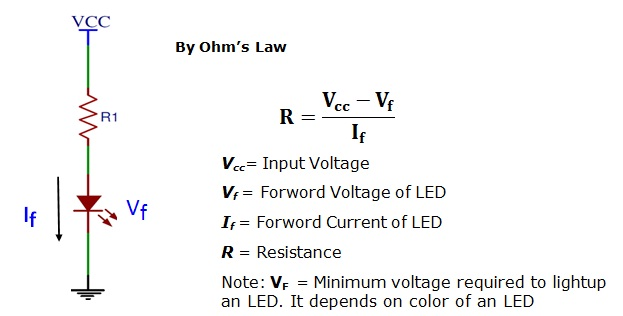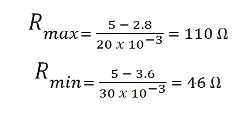Friday , August 23 2019

# What is resistor?

This post is about What is Resistor? and Why we need Resistor in Circuit. Resistor has a property of resistance (i.e. opposition to something). This property limits (controls) the flow of current to a safe value, so that other part in circuit can work properly. Simply, resistor protects circuit parts (components) from getting damage by current.

A two terminal electronic component having specific and constant electrical resistance are called as resistors. It’s a passive component (i.e. only consumes power but never generate it). Resistance (R) is measured in ‘ohm’ (Ω). Resistors used to develop a desired voltage drop in the circuit. Resistance depends on temperature change, strain and sometimes also on light illumination. The symbol of resistor is as shown below:Symbol of Resistor

#### Relationship: Voltage (V), Current (I) and Resistance (R)

With reference to Ohms law, V = I.R An example of water analogy shown in following will explain the working of resistance in circuit.Water Analogy of Resistor

#### Example Circuit: Current Limiting Resistor for LED

In this given circuit to prevent LED from damaged by excessive current, resistors (R1) is used. By connecting LED and resistor in series current can be limited to a safe value.Example Current Limiting Resistor

Lets say for example. Here we have considered data sheet of white 5mm LED. You can download it. We have

V = Vf = 2.8V (Minimum) and 3.6V (Maximum)
If = 20mA (Minimum) and 30mA (Maximum)
VR= Reverse Voltage = 5V (It is maximum possible voltage that can be applied to LED reversely (i.e. by applying reverse batterypolarity) without any damage)

According to ohm’s Law and assuming VCC= 5V, the value of resistor (R1) will becomeCalculated Value of Resistor

#### Types of Resistors

Resistors can be categorized on the basis of Value (fixed or variable), Physical appearance (shape and size), Mounting scheme (through-hole and surface mount) and some other special types.

I hope now you know what is resistor and why we need resistor in circuit. In next post we’ll learn how to calculate resistor value using color code method. Resistor is most important element in any electronic circuit, atleast I have not yet worked with any circuit without single resistor.

### About Roshankumar BhamareRoshankumar Bhamare received the Master degree in Electronics and Telecommunication engineering. He is currently a Lecturer in Engineering College. He is life member of Indian Society for Technical Education (ISTE). He is also a co-author of BINARYUPDATES.COM. His current interests include Electronics Design Automation (EDA) tools, Microcontroller based circuits.